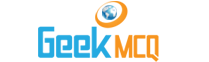# Entry Test MCQ :: Equations and Inequalities

1.  If y ≠ 0 x = 4 / y and yz = 8 then x/z = ?
 A. 0.5 B. 1 C. 2 D. 16 E. 32

2.  If the numerical values of (a-b)2 and (a+b)2 are equal then which of the following must be true?
 A. a + b = 0 B. a - b = 0 C. ab = 0 D. a = 1 / b E. a = -b

3.  If a > b > c which of the following cannot be true?
 A. b + c < a B. 2a > b + c C. 2c > a+b D. ab > bc E. a+b > 2b + c

4.  If m is an even integer and 3m<48 and 2m>24 then m = ?
 A. 12 B. 13 C. 14 D. 15 E. 16

5.  If -3 < x < 5 and -4 < y < 6 then what is the maximum possible value of y-x?
 A. 5 B. 7 C. 9 D. 10 E. 11

6.  If x2 - 9 < 0 which of the following must be true?
 A. x < -3 B. x > 3 C. x > 9 D. x < -3 or x > 3 E. ?3 < x < 3

7.  If 1/2-x/(1-x) = 1/2 then x = ?
 A. -1 B. 0 C. 1 D. 2 E. 12

8.  If y is an odd integer and 5y>35 and 2y<20 then y =?
 A. 11 B. 8 C. 9 D. 6 E. none

9.  If -9<1<11 and -10
 A. 3 B. 17 C. 21 D. 5 E. all

10.  If p is an even integer and 4p > 44 and 2p < 26 then p is :
 A. 13 B. 15 C. 12 D. 11